# RD Sharma Solutions for Class 6 Maths Chapter 19: Geometrical Constructions Exercise 19.3

Exercise 19.3 of Chapter 19 has steps explaining the bisection of a line segment based on the understanding ability of students. The primary objective of designing exercise-wise solutions is to make the subject easier for the students. While solving the problems of RD Sharma textbook for Class 6, students can download solutions’ PDF in order to gain a hold on the concepts which are covered under each exercise. Here, the students can download RD Sharma Solutions Class 6 Maths Chapter 19 Geometrical Constructions Exercise 19.3 PDF from the links which are available below.

## RD Sharma Solutions for Class 6 Maths Chapter 19: Geometrical Constructions Exercise 19.3 Download PDF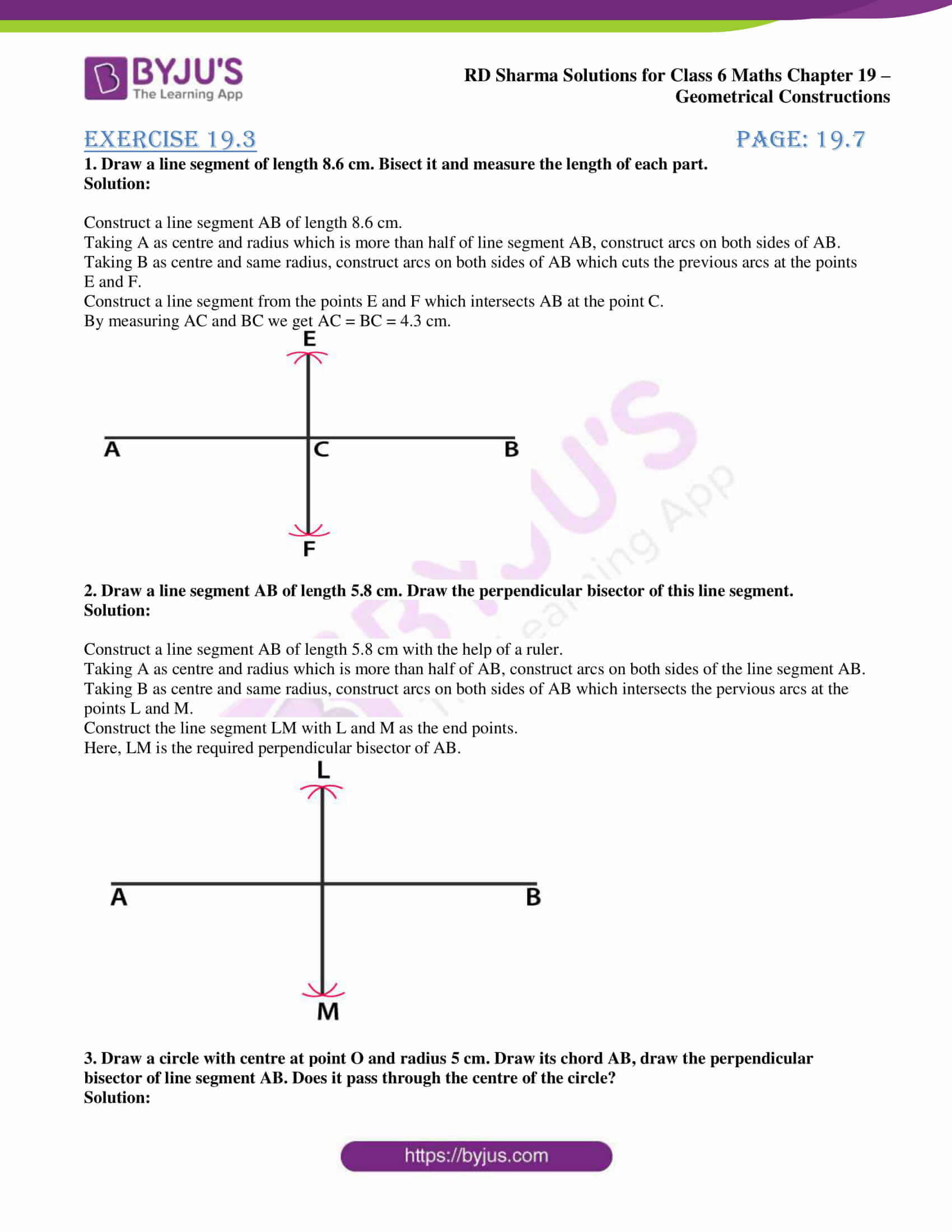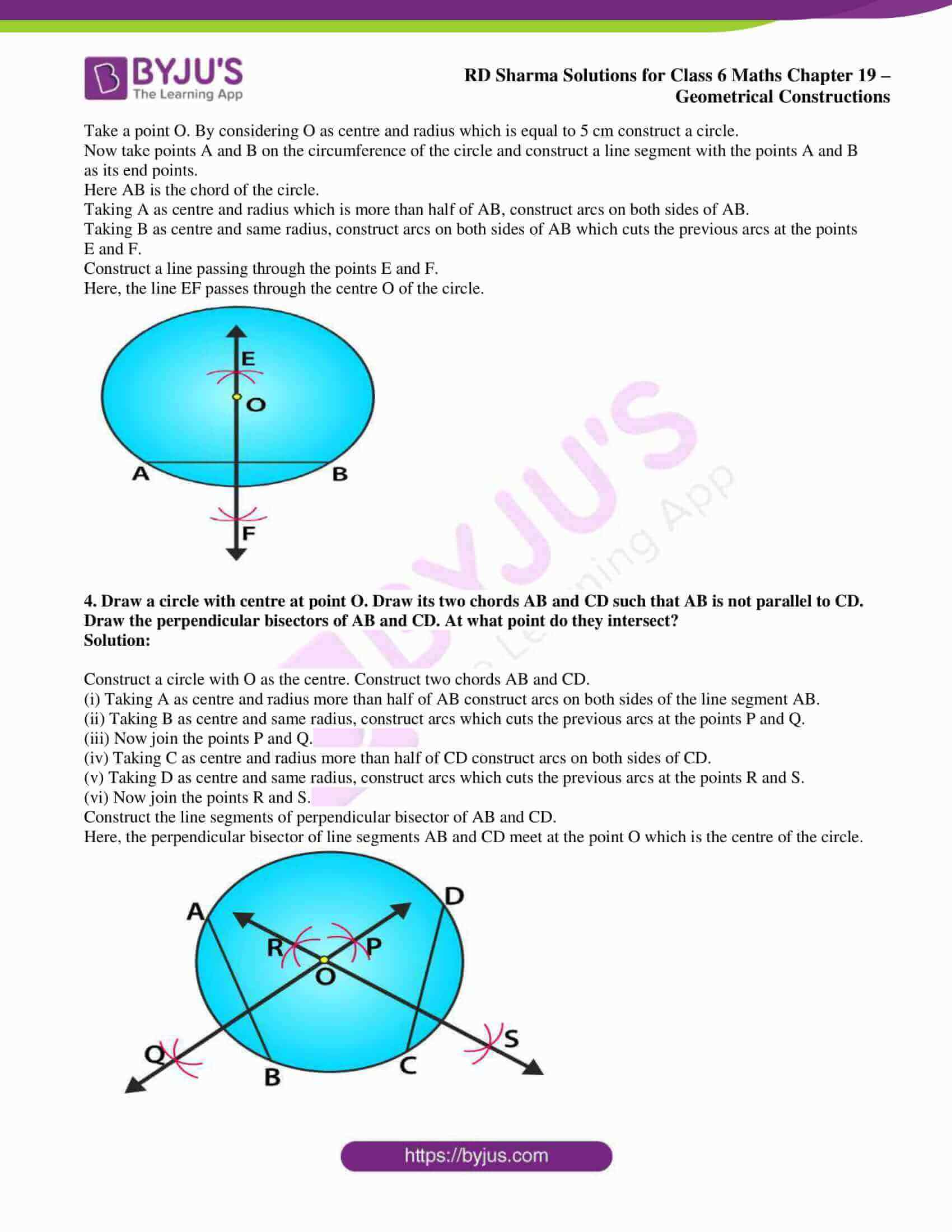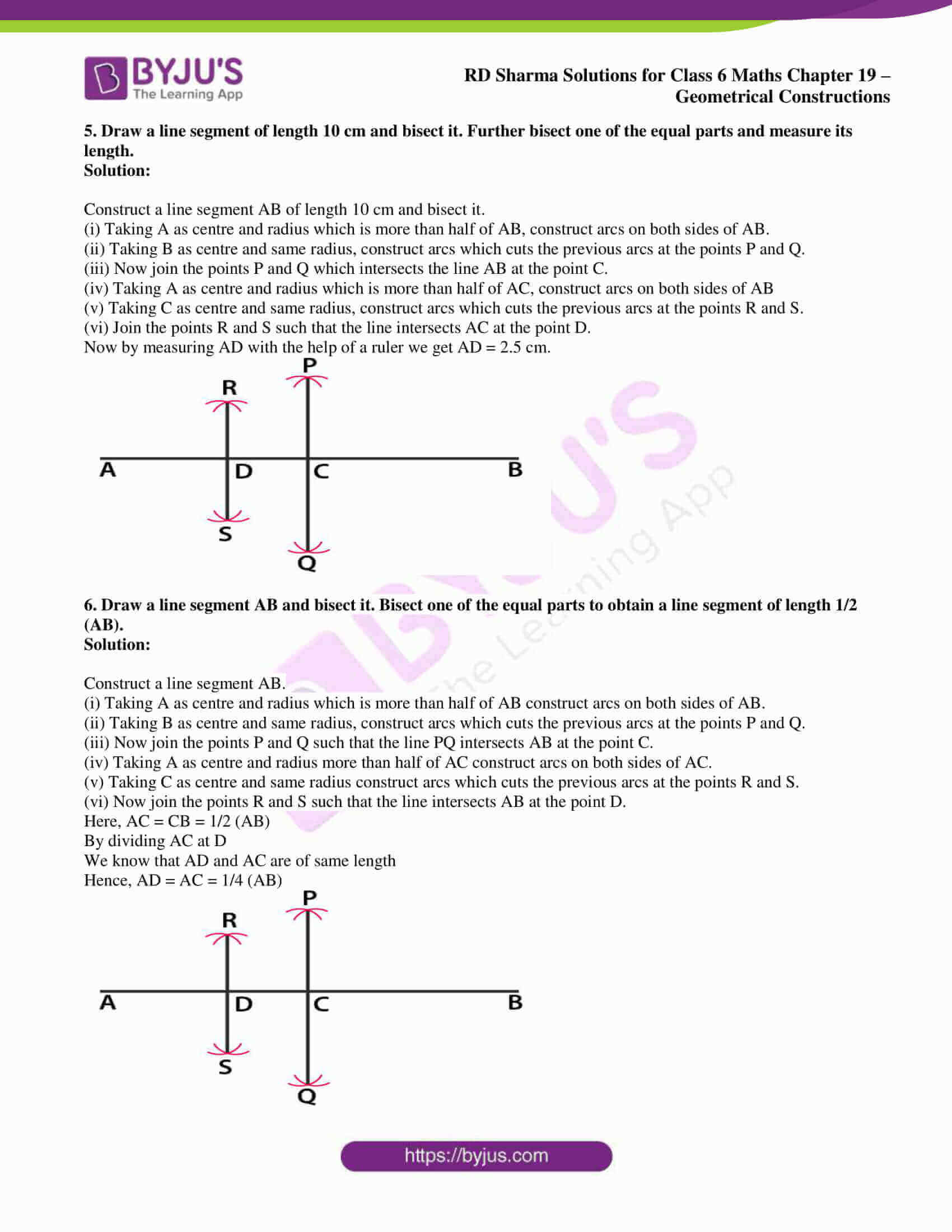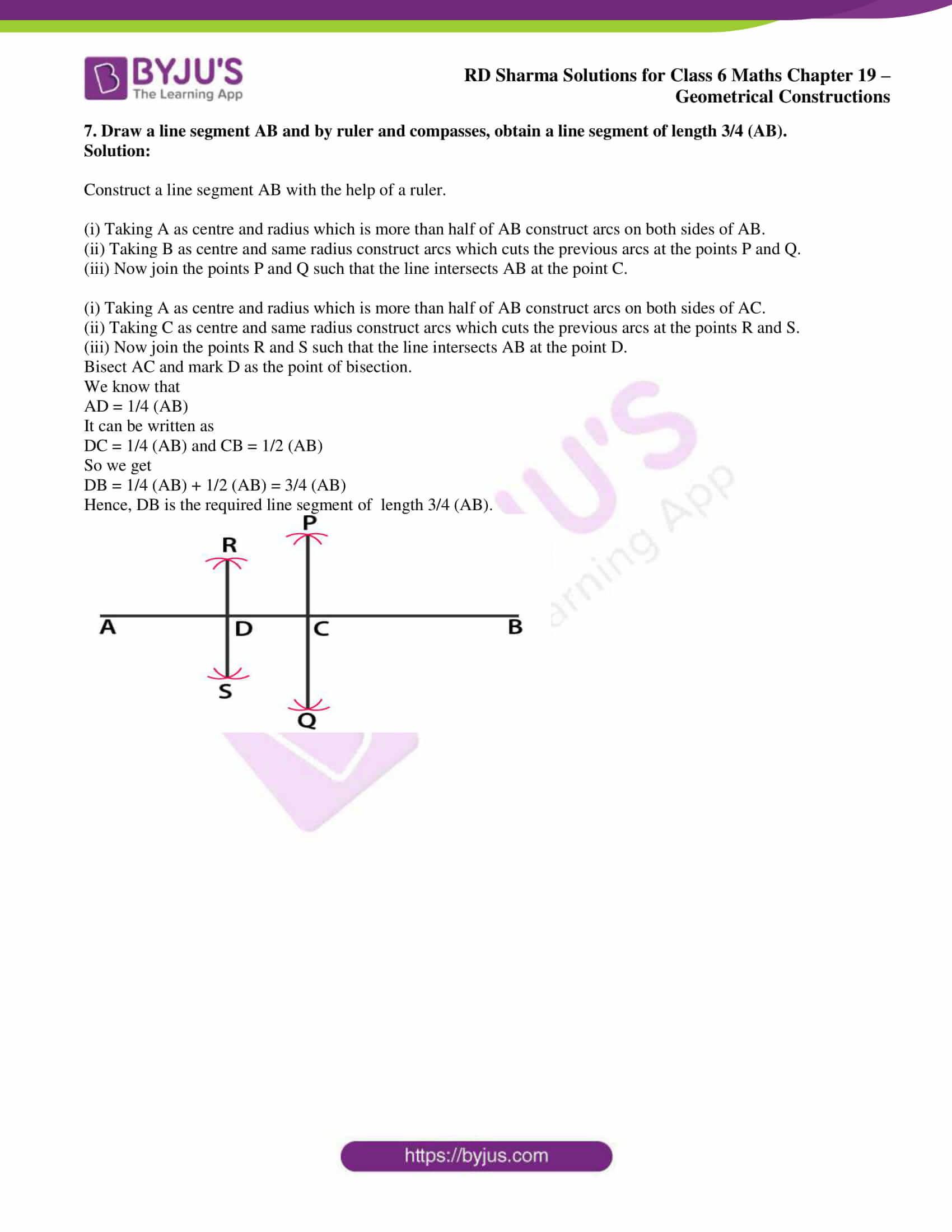### Exercise 19.3 page: 19.7

1. Draw a line segment of length 8.6 cm. Bisect it and measure the length of each part.

Solution:

Construct a line segment AB of length 8.6 cm.

Taking A as centre and radius which is more than half of line segment AB, construct arcs on both sides of AB.

Taking B as centre and same radius, construct arcs on both sides of AB which cuts the previous arcs at the points E and F.

Construct a line segment from the points E and F which intersects AB at the point C.

By measuring AC and BC we get AC = BC = 4.3 cm.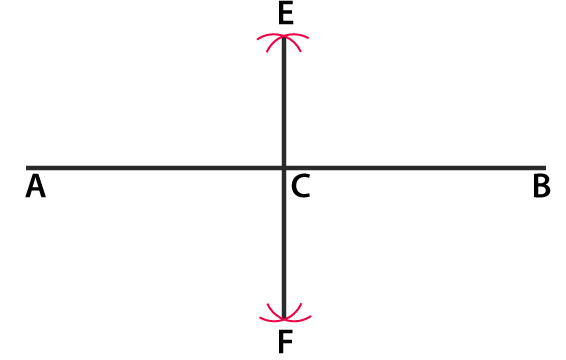2. Draw a line segment AB of length 5.8 cm. Draw the perpendicular bisector of this line segment.

Solution:

Construct a line segment AB of length 5.8 cm with the help of a ruler.

Taking A as centre and radius which is more than half of AB, construct arcs on both sides of the line segment AB.

Taking B as centre and same radius, construct arcs on both sides of AB which intersects the pervious arcs at the points L and M.

Construct the line segment LM with L and M as the end points.

Here, LM is the required perpendicular bisector of AB.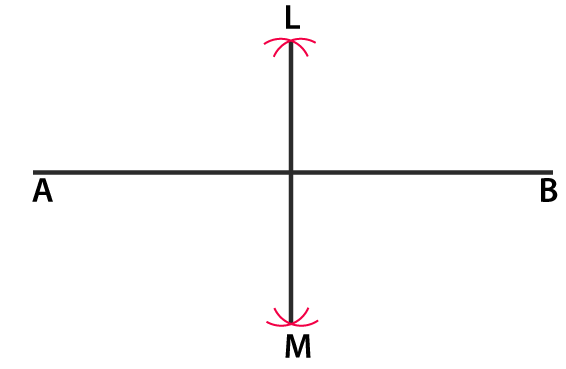3. Draw a circle with centre at point O and radius 5 cm. Draw its chord AB, draw the perpendicular bisector of line segment AB. Does it pass through the centre of the circle?

Solution:

Take a point O. By considering O as centre and radius which is equal to 5 cm construct a circle.

Now take points A and B on the circumference of the circle and construct a line segment with the points A and B as its end points.

Here AB is the chord of the circle.

Taking A as centre and radius which is more than half of AB, construct arcs on both sides of AB.

Taking B as centre and same radius, construct arcs on both sides of AB which cuts the previous arcs at the points E and F.

Construct a line passing through the points E and F.

Here, the line EF passes through the centre O of the circle.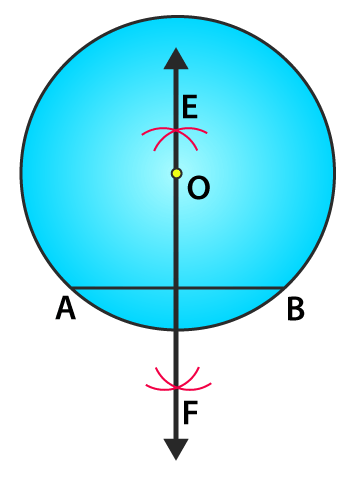4. Draw a circle with centre at point O. Draw its two chords AB and CD such that AB is not parallel to CD. Draw the perpendicular bisectors of AB and CD. At what point do they intersect?

Solution:

Construct a circle with O as the centre. Construct two chords AB and CD.

(i) Taking A as centre and radius more than half of AB construct arcs on both sides of the line segment AB.

(ii) Taking B as centre and same radius, construct arcs which cuts the previous arcs at the points P and Q.

(iii) Now join the points P and Q.

(iv) Taking C as centre and radius more than half of CD construct arcs on both sides of CD.

(v) Taking D as centre and same radius, construct arcs which cuts the previous arcs at the points R and S.

(vi) Now join the points R and S.

Construct the line segments of perpendicular bisector of AB and CD.

Here, the perpendicular bisector of line segments AB and CD meet at the point O which is the centre of the circle.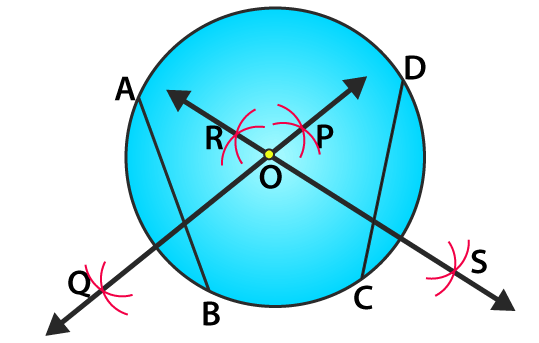5. Draw a line segment of length 10 cm and bisect it. Further bisect one of the equal parts and measure its length.

Solution:

Construct a line segment AB of length 10 cm and bisect it.

(i) Taking A as centre and radius which is more than half of AB, construct arcs on both sides of AB.

(ii) Taking B as centre and same radius, construct arcs which cuts the previous arcs at the points P and Q.

(iii) Now join the points P and Q which intersects the line AB at the point C.

(iv) Taking A as centre and radius which is more than half of AC, construct arcs on both sides of AB

(v) Taking C as centre and same radius, construct arcs which cuts the previous arcs at the points R and S.

(vi) Join the points R and S such that the line intersects AC at the point D.

Now by measuring AD with the help of a ruler we get AD = 2.5 cm.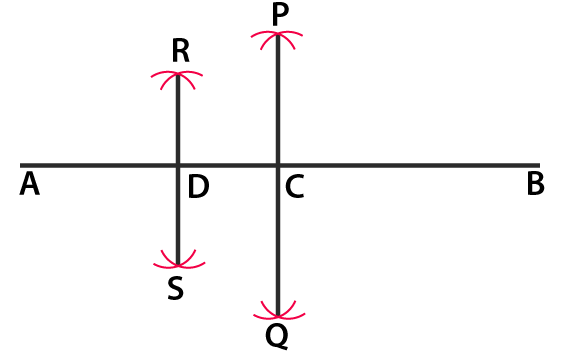6. Draw a line segment AB and bisect it. Bisect one of the equal parts to obtain a line segment of length 1/2 (AB).

Solution:

Construct a line segment AB.

(i) Taking A as centre and radius which is more than half of AB construct arcs on both sides of AB.

(ii) Taking B as centre and same radius, construct arcs which cuts the previous arcs at the points P and Q.

(iii) Now join the points P and Q such that the line PQ intersects AB at the point C.

(iv) Taking A as centre and radius more than half of AC construct arcs on both sides of AC.

(v) Taking C as centre and same radius construct arcs which cuts the previous arcs at the points R and S.

(vi) Now join the points R and S such that the line intersects AB at the point D.

Here, AC = CB = 1/2 (AB)

By dividing AC at D

We know that AD and AC are of same length

Hence, AD = AC = 1/4 (AB)7. Draw a line segment AB and by ruler and compasses, obtain a line segment of length 3/4 (AB).

Solution:

Construct a line segment AB with the help of a ruler.

(i) Taking A as centre and radius which is more than half of AB construct arcs on both sides of AB.

(ii) Taking B as centre and same radius construct arcs which cuts the previous arcs at the points P and Q.

(iii) Now join the points P and Q such that the line intersects AB at the point C.

(i) Taking A as centre and radius which is more than half of AB construct arcs on both sides of AC.

(ii) Taking C as centre and same radius construct arcs which cuts the previous arcs at the points R and S.

(iii) Now join the points R and S such that the line intersects AB at the point D.

Bisect AC and mark D as the point of bisection.

We know that